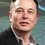# Help needed (Golden Ratio?)man

A staircase has n steps. A man climbs either one step or two steps at a time .Prove that the number of ways in which he can climb up the staircase , starting from the bottom , is

$\huge{\frac{1}{\sqrt{5}}[(\frac{ 1 + \sqrt{5}}{2})^{n + 1} - (\frac{ 1 - \sqrt{5}}{2})^{n + 1}]}$ $n \geq 1$Note by U Z
6 years, 8 months ago

This discussion board is a place to discuss our Daily Challenges and the math and science related to those challenges. Explanations are more than just a solution — they should explain the steps and thinking strategies that you used to obtain the solution. Comments should further the discussion of math and science.

When posting on Brilliant:

• Use the emojis to react to an explanation, whether you're congratulating a job well done , or just really confused .
• Ask specific questions about the challenge or the steps in somebody's explanation. Well-posed questions can add a lot to the discussion, but posting "I don't understand!" doesn't help anyone.
• Try to contribute something new to the discussion, whether it is an extension, generalization or other idea related to the challenge.

MarkdownAppears as
*italics* or _italics_ italics
**bold** or __bold__ bold
- bulleted- list
• bulleted
• list
1. numbered2. list
1. numbered
2. list
Note: you must add a full line of space before and after lists for them to show up correctly
paragraph 1paragraph 2

paragraph 1

paragraph 2

[example link](https://brilliant.org)example link
> This is a quote
This is a quote
    # I indented these lines
# 4 spaces, and now they show
# up as a code block.

print "hello world"
# I indented these lines
# 4 spaces, and now they show
# up as a code block.

print "hello world"
MathAppears as
Remember to wrap math in $$ ... $$ or $ ... $ to ensure proper formatting.
2 \times 3 $2 \times 3$
2^{34} $2^{34}$
a_{i-1} $a_{i-1}$
\frac{2}{3} $\frac{2}{3}$
\sqrt{2} $\sqrt{2}$
\sum_{i=1}^3 $\sum_{i=1}^3$
\sin \theta $\sin \theta$
\boxed{123} $\boxed{123}$

Sort by:

Let $T_n$ be the number of steps required to climb a staircase with $n$ steps.

$T_1=1$

$T_2=2$

For $n\geq3$, as @Michael Mendrin mentioned, we can start with one step, and the rest of it are $T_{n-1}$ steps. If we start on two steps, the rest of it are $T_{n-2}$ steps. Now we have a conclusion that

$T_n=T_{n-1}+T_{n-2}$

This is actually the Fibonacci Sequence, but we have to shift it to the right, since it starts with $1,2,...$ instead of the typical $1,1,2,...$. Hence,

$T_n=F_{n+1}$

- 6 years, 8 months ago

- 6 years, 8 months ago

Well, let's see, let's say there's $a$ ways to climb up $n-2$ steps, and $b$ ways to climb up $n-1$ steps, so if we backtrack from the $n$th step either $1$ or $2$ steps, then those would be the number of ways to get to those previous steps. Hence, the number of ways to get to the $n$th step is $a+b$, and we're looking at Fibonacci numbers. The formula give was a dead giveaway to the answer to this one. Thanks for the hint!

Addendum: If one wonders if starting at $n-2$ steps, there are actually $2$ ways to get to the $n$th step, in fact the $1+1$ steps to the $n$th step is already part of counting the ways up to and through the $n-1$th step. So, it's still a string of honest Fibonacci numbers.

- 6 years, 8 months ago

The number of ways is just the fibonacci sequence. You can see this by noting the number of ways to climb n steps is equal to the number of ways where the second to last step gets you up n-2 steps, plus the number of ways where the second to last step gets you up n-1 steps. If s(n) represents the number of ways to climb up n steps, then it follows that s(n) + s(n+1) = s(n+2). Since s(1) = 1 and s(2) = 2 by inspection, this is just the fibonacci sequence shifted by 1, as expressed in the formula.

- 6 years, 8 months ago

We can notice that this looks similar to Binet’s Theorem, which is an explicit formula to give the nth number in the fibonacci sequence. In this case, it gives the (n+1)th term. The Fibonacci sequence is 1 1 2 3 5 8… while this sequence is 1 2 3 5 8… because the number of ways to get to each step adds up. This sequence is just the Fibonacci sequence with the first two terms as 1 and 2. QED

- 6 years, 8 months ago

- 6 years, 8 months ago

- 6 years, 8 months ago

As Christopher and Michael pointed out, you should have tried generating the first few terms of the series. You'll find it's a fibonacci series and if you are not familiar with the formula, look it up. :)

- 6 years, 8 months ago

Where's n in that expression??

- 6 years, 8 months ago

O sorry thank you

- 6 years, 8 months ago

Hint:- Use recurrence relations. Define $T_n$ as the number of ways to climb a staircase with $n$ steps. Shows its relation with $T_{n-1}$ and $T_{n-2}$. You should come up with a familiar relation.

- 6 years, 8 months ago

I think it has got something to do with Fibonacci numbers cause the expression gives the $n+1$ th Fibonacci number.

- 6 years, 8 months ago

I encountered a similar question in the NMTC screening test this year.

- 6 years, 8 months ago